Understanding Carboxylic Acids and Derivatives

Understanding Carboxylic Acids and Derivatives

Key Terms

• Carboxylic acid
• Carboxyl group
• Acyl chloride
• Acid anhydride
• Carboxylation
• Grignard reagent
• Organometallic compound
• Nitrile
• Acid-catalyzed esterification

Objectives

• Recognize and name carboxylic acids and some of their chief derivatives
• Study two synthesis reactions for carboxylic acids
• Describe the mechanism for a representative reaction involving carboxylic acids

In this article, we will briefly introduce carboxylic acids and some of their derivative compounds, beginning with the nomenclature followed by some representative reactions involving these chemicals. As with some of the compounds, carboxylic acids combine multiple functional groups (carbonyl and hydroxyl groups in the case of a generic carboxylic acid).

A carboxylic acid has the general form shown below, where R is a substituent alkyl group. The functional group attached to the R alkyl group is called a carboxyl group. Several example carboxylic acids and their names (systematic and common) follow.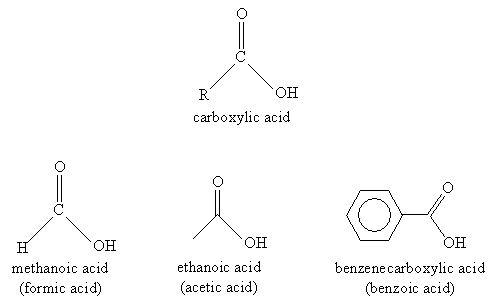A number of similar molecules are considered derivatives of carboxylic acids. These compounds involve substitution of the hydroxyl group with another group, such as a chlorine atom. These derivatives are all linked by their combination of an acyl group with an electronegative element in the substituent group. The basic carboxylic acid derivatives we will consider are acyl chlorides, esters, and acid anhydrides. The general structure of each compound is shown below, where R1 and R2 are alkyl groups.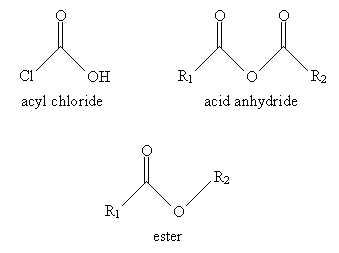Nomenclature

Nomenclature for carboxylic acids is relatively simple. You can probably guess from the examples shown above that carboxylic acids involving carbon chains are simply named by removing the -e ending from the longest carbon chain (including the carbonyl group) and adding -oic acid. Thus, for instance, the molecule below would be 4-methylhexanoic acid. Of course, the carbon atom in the carboxyl group is assumed to be at position 1.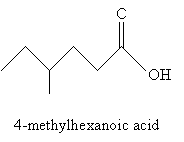When the carboxyl group is attached to a ring structure, such as benzene, the full ring name is simply appended with -carboxylic acid, as in the above case of benzenecarboxylic acid. Another example is 3-chlorocyclohexanecarboxylic acid, which is shown below.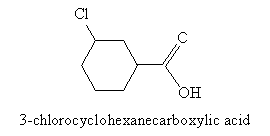Practice Problem: Identify the systematic IUPAC name for the following molecule.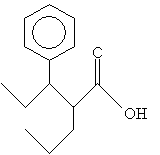Solution: First, identify the longest carbon chain that includes the carbon atom in the carboxyl group. (Here, you have two choices-to simplify the name, choose the carbon chain that includes the phenyl group as a substituent.) Then number the chain and follow the usual rules of IUPAC nomenclature, ending the name with -oic acid.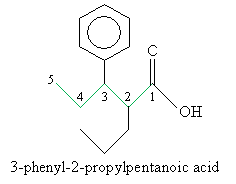For derivatives of carboxylic acids, the nomenclature is similar but with a few slight variations. In the case of acyl chlorides, use the ending -yl chloride instead of -oic acid. Thus, for instance: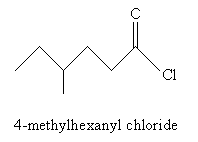For acid anhydrides, use the suffix -oic for each substituent alkyl group (R1 and R2 in the generic example above), followed by anhydride. If R1 and R2 are the same, you need only use one of them in the name. Thus, for example: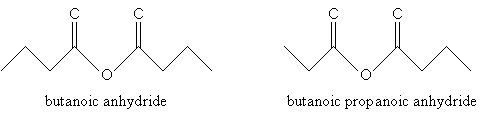Practice Problem: Determine the IUPAC names for the two molecules below.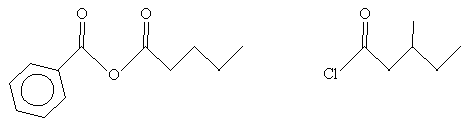Solution: In the case of the molecule on the left (an acid anhydride), note that the two substituent alkyl groups are a benzene ring (left) and a butane chain. The corresponding names are benzoic and pentanoic, respectively. For the molecule on the right (an acyl chloride), the carbon chain (including the carbon in the carbonyl group) is a methyl-substituted pentyl group. Thus, the name for each molecule is shown below.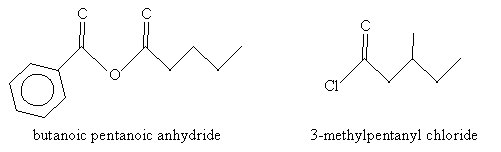Acidity of Carboxylic Acids

Note that α hydrogens (relative to the carbonyl group) are fairly acidic. The hydrogen in the carboxyl group is also relatively acidic. Consider acetic acid, for example. When the hydrogen atom (proton) is abstracted from the carboxyl group, the resulting form has two resonance structures, increasing its stability.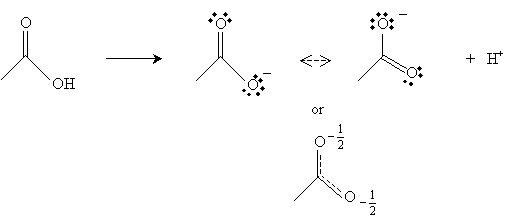Furthermore, the carbon in the carbonyl group has a net positive charge because the doubly bonded oxygen tends to draw the electrons; thus, this positive charge somewhat balances the negative charge of the other oxygen in the carboxyl group.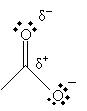These characteristics make the hydrogen in the hydroxl group relatively acidic for an organic compound.

Synthesis of Carboxylic Reactions

We present two synthesis reactions for carboxylic acids. The first is carboxylation of a Grignard reagent. A Grignard reagent is an organometallic compound containing magnesium (Mg). (As the name indicates, an organometallic compound is a chemical containing a metal component and an organic component.) In a diethyl ether solvent, magnesium and an alkyl halide (RX) form a Grignard reagent (RMgX). In the presence of carbon dioxide and acidic conditions (H3O+), the Grignard reagent forms a carboxylic acid. The reaction is summarized below for the case of benzenecarboxylic acid.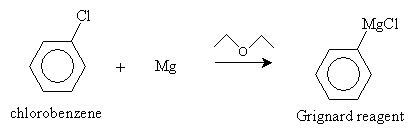The next step in the reaction is a reaction of the Grignard reagent with carbon dioxide.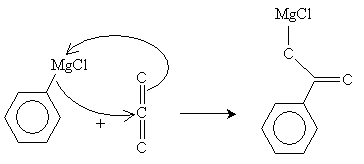Finally, in the presence of H3O+ (acidic conditions), benzenecarboxylic acid is formed.A second type of synthesis reaction is hydrolysis of a nitrile. A nitrile is an organic compound with a cyanide (CN-) group; an example is shown below.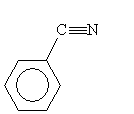Consider the nitrile below. In an acidic solution and with added heat, a carboxylic acid is formed.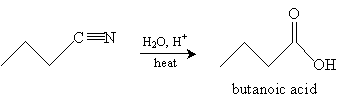Practice Problem: What is the organic product of the reaction below?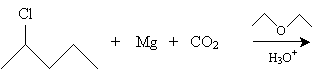Solution: This reaction creates a Grignard reagent (shown below) that reacts with carbon dioxide in acidic conditions to produce a carboxylic acid.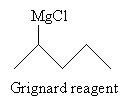Following the reaction mechanism discussed above, the product in this case is 2-methylpentanoic acid.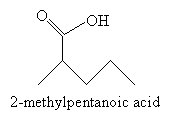Reactions of Carboxylic Acids

We consider just one representative reaction of a carboxylic acid: acid-catalyzed esterification. In this reaction, an alcohol interacts with a carboxylic acid in an acidic environment to yield an ester and water. The overall reaction for acetic acid and methanol is shown below.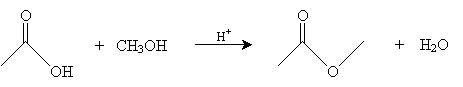The mechanism for this reaction is as follows. First, the methanol molecule gains a proton from the acid present in the reaction environment, forming CH3OH2+, which is shown below.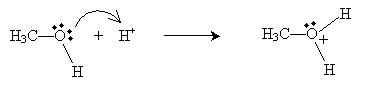This new cation then donates a proton to the doubly bonded oxygen in acetic acid as follows.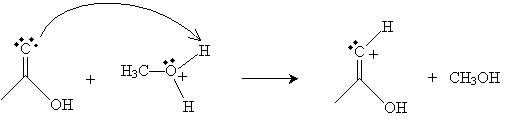Next, the oxygen in the methanol molecule attacks the carbon in the carboxyl group.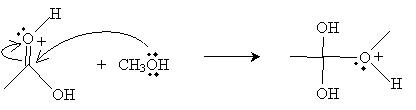Another methanol molecule then accepts a proton from the positively charged oxygen in the product above.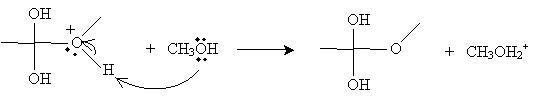The next step involves the methanol-based cation above donating a proton to one of the hydroxyl groups in the intermediate form.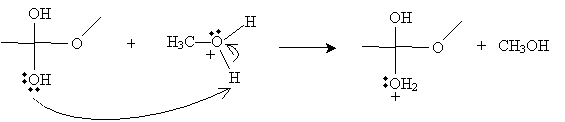Next, the cation intermediate dissociates a water molecule as follows.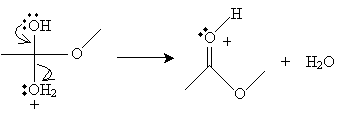Finally, a methanol molecule accepts a proton from the cation, completing the reaction.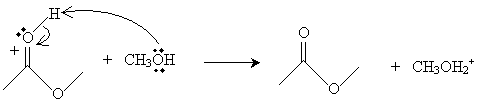The result of this somewhat convoluted process is an ester-in this case, methyl ethanoate (or methyl acetate).

Practice Problem: Butanoic acid and propanol combine under acidic conditions to form what products?

Solution: The overall reaction of a carboxylic acid (butanoic acid, in this case) and an alcohol (propanol) is the following: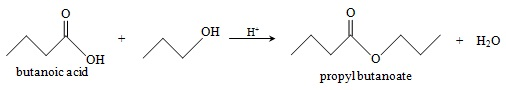The reaction follows the mechanism described earlier. The products are propyl butanoate and water.

Explore